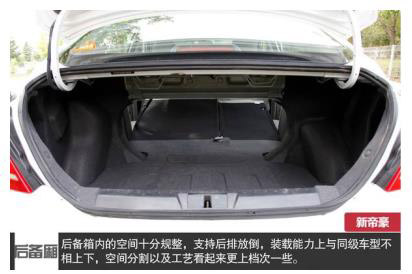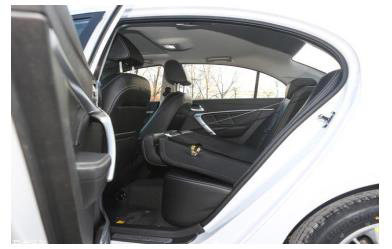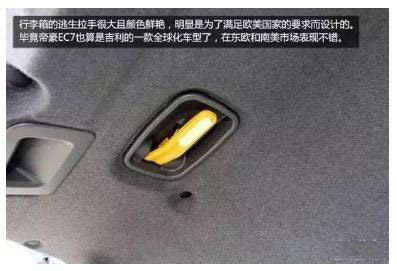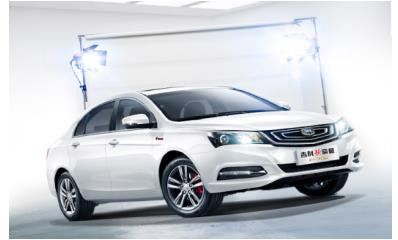## 据说 具备这个功能的汽车安全才有保障

2017年06月06日 10:13 来源：www.chextx.com 超过：次关注

在电影《神奇女侠》中，男主开飞机掉进了天堂岛的海里，被困驾驶舱中无法脱身，眼看自己肺活量的极限就要到了。碰巧的是，这一幕被众神之神的宙斯和亚马逊女王生下的女儿（女主）在海边放风时撞见了。作为天神，拯救人类是她的天职（也可以说拯救自己第一个见到的男人是生命中的小确幸），最终，女神毫不犹豫地跳进海里，救出了落水的飞行员，然后两个人（应该是一个神、一个人）一起开始了一段紧张又刺激的生活……

日常生活中，想必大家不会有开飞机掉水里的体验。开车掉水里的经验虽说也只是偶尔在新闻里看到，但是万一真要是自己摊上这事，没有电影里的女神相救可咋办？！不用担心，没有女神，也可以“逃出升天”——吉利新帝豪百万款标配的后备箱逃生功能，让你即使身处困境，也能从容应对，带来不可思议的安全保护体验。

关于后备箱逃生功能，更多时候是为了应对突发事件，平常几乎用不到，也正是这样，吉利帝豪一贯的安全理念和产品性能才凸显出来：防微杜渐，有备无患，才是安全的最好境界！必须要强调的是，大部分十多万合资车都少有的后备箱逃生功能，在帝豪这里可是标配，连同标配了的后排座椅放倒功能，帝豪为乘车人员构筑起了一道坚固的安全防线。下面，就来看看帝豪的后备箱逃生功能都在哪些使用场景中为用户带来优质体验：

1.后备箱放置较长物品。作为家轿，放置家用物品是必须的，一般的物品还好，较长的就不好放置了，这时候，帝豪的后排放倒功能和后备箱逃生功能就能发挥作用，完美解决这些日常的家用需求。

2.当防盗系统失效，或者当发生交通事故，车辆电控失败等情况，导致后备箱用正常方式无法打开时，可以通过后备箱逃生功能逃出车内，再用机械钥匙打开车门（以往遇到这种状况只能砸玻璃，现在有了帝豪的后备箱逃生功能，简直可以省下了不少玻璃钱）。

3.遭遇恶劣环境（雨天、冰冻天，沙尘暴、稀泥等）。出行遇到恶劣天气和环境，需要的物品（如雨伞、雨鞋、手电、工具和水等）却在后备箱时，别的车可能不得不让人下车去取，在帝豪这里，后排座椅放倒和后备箱逃生两大功能让你不用下车，就能轻松get到自己所需。

前面的情况，还是算是应对一些日常出行问题，下面两种情况，才真正显示出帝豪后备箱逃生功能关键时刻的生命守护能力。1.小孩被误锁车内。汽车的普及，在越来越多家庭带来便利同时，也引发了不少悲剧，比如误锁儿童在车内，最终造成惨剧。而有了帝豪后备箱逃生功能，即使父母来不及救援，也可以隔空指导孩子通过后备箱逃出车外，脱离危险！

2.汽车不慎掉入河里。越是极少出现的紧急情况，相应应对的功能就显得越为重要。落水后水压会导致电控失败和车门无法打开，由于车头比车尾重，这时车头向下车尾朝上，非常危险。此时，当事人就可以通过帝豪后备箱逃生功能脱离危险。在帝豪这里，后备箱可不只是容器，还是带来希望的紧急助手（友情提示：后备箱逃生功能虽然好用，可不能故意开到河里实验！）。综上所述，后备箱逃生功能堪称是汽车界的良心配置，全方位的考虑了车主的行车安全，同时也提升了车辆的可玩性。帝豪作为国民座驾，标配了后备箱逃生功能和后排座椅放倒功能，不仅让用户体验到越级的配置，更为用户各种用车的场景提供了人性化的呵护，让大家再次感受到“最懂国人的车”的风范！

#### 相关文章

﻿
• 快速找车
• 选择品牌
• 选择品牌
• A  奥迪
• A  阿斯顿·马丁
• A  阿尔法·罗密欧
• B  宝沃
• B  布加迪
• B  巴博斯
• B  保时捷
• B  宾利
• B  奔驰
• B  宝马
• B  本田
• B  别克
• B  标致
• B  比亚迪
• B  宝骏
• B  北汽制造
• B  北汽新能源
• B  北汽幻速
• B  北汽威旺
• B  北京汽车
• B  奔腾
• B  北汽绅宝
• B  北汽昌河
• C  长安欧尚
• C  长安
• C  长安凯程
• C  长城
• D  大众
• D  道奇
• D  DS
• D  东南
• D  东风风神
• D  东风风行
• D  东风小康
• D  东风风度
• D  东风
• F  福特
• F  丰田
• F  菲亚特
• F  法拉利
• F  福田
• F  福迪
• F  福汽启腾
• G  观致
• G  广汽传祺
• G  广汽吉奥
• G  GMC
• H  红旗
• H  汉腾汽车
• H  哈弗
• H  哈飞
• H  海格
• H  海马
• H  华颂
• H  黄海
• H  华泰
• H  恒天
• J  捷途
• J  几何汽车
• J  捷达
• J  吉利汽车
• J  捷豹
• J  Jeep
• J  江淮
• J  江铃
• J  金杯
• J  九龙
• J  金旅
• K  凯翼
• K  凯迪拉克
• K  克莱斯勒
• K  科尼塞克
• K  卡威
• K  开瑞
• L  路虎
• L  林肯
• L  劳斯莱斯
• L  兰博基尼
• L  雷克萨斯
• L  铃木
• L  领克
• L  雷诺
• L  理念
• L  力帆
• L  莲花汽车
• L  猎豹
• L  路特斯
• L  陆风
• M  马自达
• M  MG
• M  MINI
• M  玛莎拉蒂
• M  摩根
• M  迈凯轮
• N  纳智捷
• O  欧拉
• O  欧宝
• O  讴歌
• O  欧朗
• Q  奇瑞
• Q  起亚
• Q  启辰
• R  日产
• R  荣威
• R  瑞麒
• S  SERES赛力斯
• S  三菱
• S  斯威汽车
• S  萨博
• S  smart
• S  斯柯达
• S  斯巴鲁
• S  思铭
• S  双龙
• S  上汽大通
• S  双环
• T  特斯拉
• T  腾势
• W  蔚来
• W  沃尔沃
• W  WEY
• W  五菱汽车
• W  五十铃
• W  威兹曼
• W  威麟
• X  现代
• X  雪佛兰
• X  星途
• X  雪铁龙
• X  小鹏汽车
• X  西雅特
• Y  一汽
• Y  英菲尼迪
• Y  英致
• Y  依维柯
• Y  野马汽车
• Y  永源
• Z  众泰
• Z  中华
• Z  中兴
• Z  知豆
• 选择车系
• 选择车系
• 车型对比
• 选择品牌
• 选择品牌
• A  奥迪
• A  阿斯顿·马丁
• A  阿尔法·罗密欧
• B  宝沃
• B  布加迪
• B  巴博斯
• B  保时捷
• B  宾利
• B  奔驰
• B  宝马
• B  本田
• B  别克
• B  标致
• B  比亚迪
• B  宝骏
• B  北汽制造
• B  北汽新能源
• B  北汽幻速
• B  北汽威旺
• B  北京汽车
• B  奔腾
• B  北汽绅宝
• B  北汽昌河
• C  长安欧尚
• C  长安
• C  长安凯程
• C  长城
• D  大众
• D  道奇
• D  DS
• D  东南
• D  东风风神
• D  东风风行
• D  东风小康
• D  东风风度
• D  东风
• F  福特
• F  丰田
• F  菲亚特
• F  法拉利
• F  福田
• F  福迪
• F  福汽启腾
• G  观致
• G  广汽传祺
• G  广汽吉奥
• G  GMC
• H  红旗
• H  汉腾汽车
• H  哈弗
• H  哈飞
• H  海格
• H  海马
• H  华颂
• H  黄海
• H  华泰
• H  恒天
• J  捷途
• J  几何汽车
• J  捷达
• J  吉利汽车
• J  捷豹
• J  Jeep
• J  江淮
• J  江铃
• J  金杯
• J  九龙
• J  金旅
• K  凯翼
• K  凯迪拉克
• K  克莱斯勒
• K  科尼塞克
• K  卡威
• K  开瑞
• L  路虎
• L  林肯
• L  劳斯莱斯
• L  兰博基尼
• L  雷克萨斯
• L  铃木
• L  领克
• L  雷诺
• L  理念
• L  力帆
• L  莲花汽车
• L  猎豹
• L  路特斯
• L  陆风
• M  马自达
• M  MG
• M  MINI
• M  玛莎拉蒂
• M  摩根
• M  迈凯轮
• N  纳智捷
• O  欧拉
• O  欧宝
• O  讴歌
• O  欧朗
• Q  奇瑞
• Q  起亚
• Q  启辰
• R  日产
• R  荣威
• R  瑞麒
• S  SERES赛力斯
• S  三菱
• S  斯威汽车
• S  萨博
• S  smart
• S  斯柯达
• S  斯巴鲁
• S  思铭
• S  双龙
• S  上汽大通
• S  双环
• T  特斯拉
• T  腾势
• W  蔚来
• W  沃尔沃
• W  WEY
• W  五菱汽车
• W  五十铃
• W  威兹曼
• W  威麟
• X  现代
• X  雪佛兰
• X  星途
• X  雪铁龙
• X  小鹏汽车
• X  西雅特
• Y  一汽
• Y  英菲尼迪
• Y  英致
• Y  依维柯
• Y  野马汽车
• Y  永源
• Z  众泰
• Z  中华
• Z  中兴
• Z  知豆
• 选择车系
• 选择车系
• 选择车型
• 选择车型
• 意见反馈